# Functional Skills Maths Area perimeter and volume December

• Slides: 15
Download presentation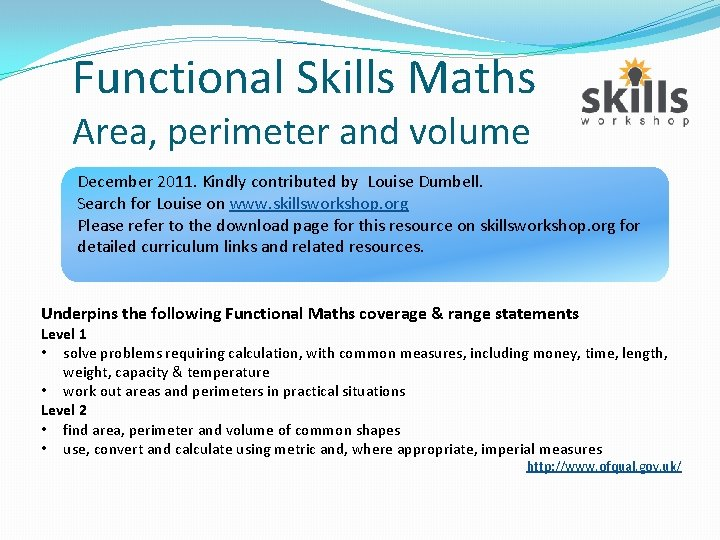Functional Skills Maths Area, perimeter and volume December 2011. Kindly contributed by Louise Dumbell. Search for Louise on www. skillsworkshop. org Please refer to the download page for this resource on skillsworkshop. org for detailed curriculum links and related resources. Underpins the following Functional Maths coverage & range statements Level 1 • solve problems requiring calculation, with common measures, including money, time, length, weight, capacity & temperature • work out areas and perimeters in practical situations Level 2 • find area, perimeter and volume of common shapes • use, convert and calculate using metric and, where appropriate, imperial measures http: //www. ofqual. gov. uk/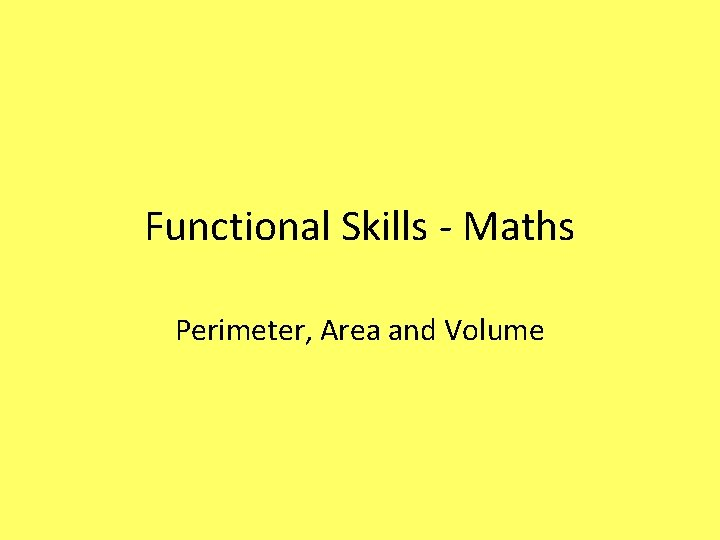Functional Skills - Maths Perimeter, Area and Volume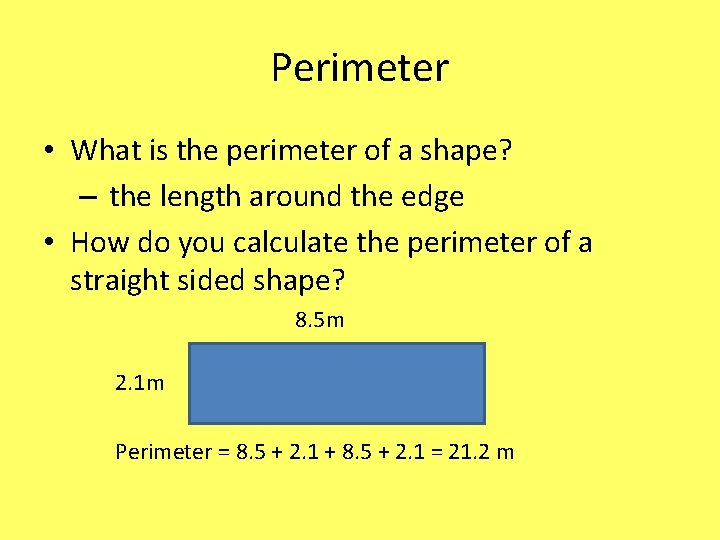Perimeter • What is the perimeter of a shape? – the length around the edge • How do you calculate the perimeter of a straight sided shape? 8. 5 m 2. 1 m Perimeter = 8. 5 + 2. 1 + 8. 5 + 2. 1 = 21. 2 m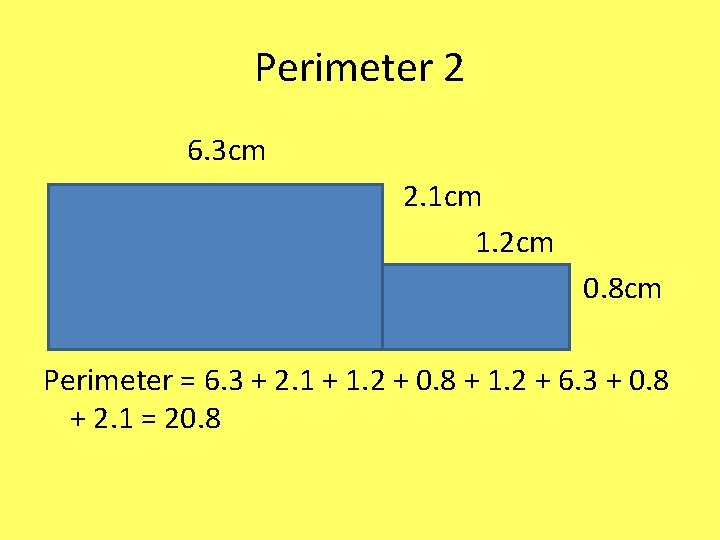Perimeter 2 6. 3 cm 2. 1 cm 1. 2 cm 0. 8 cm Perimeter = 6. 3 + 2. 1 + 1. 2 + 0. 8 + 1. 2 + 6. 3 + 0. 8 + 2. 1 = 20. 8Perimeter 3 • What is the name we use for the perimeter of a circle? Circumference • How do we calculate the circumference of a circle? 2 x radius x π radius = distance from centre to the edge of a circle π = 3. 142Perimeter 4 • Radius = 5. 2 mm • Perimeter = 2 x 5. 2 x π = 32. 67 mm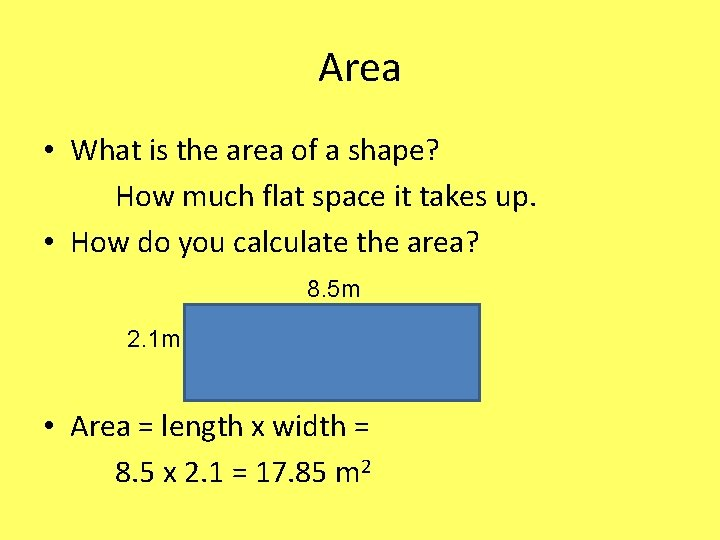Area • What is the area of a shape? How much flat space it takes up. • How do you calculate the area? 8. 5 m 2. 1 m • Area = length x width = 8. 5 x 2. 1 = 17. 85 m 2Area 2 6. 3 cm 2. 1 cm 1. 2 cm 0. 8 cm Area = (6. 3 x (2. 1+0. 8)) + (1. 2 x 0. 8) =(6. 3 x 2. 9) + (1. 2 x 0. 8) = 18. 27 + 0. 96 = 19. 23 cm 2Area 3 • What is the area of a circle? π x (radius)2 Or π x radiusArea 4 • Radius = 5. 2 mm • Area = π x 5. 22 = 3. 142 x 5. 2 = 84. 95 mm 2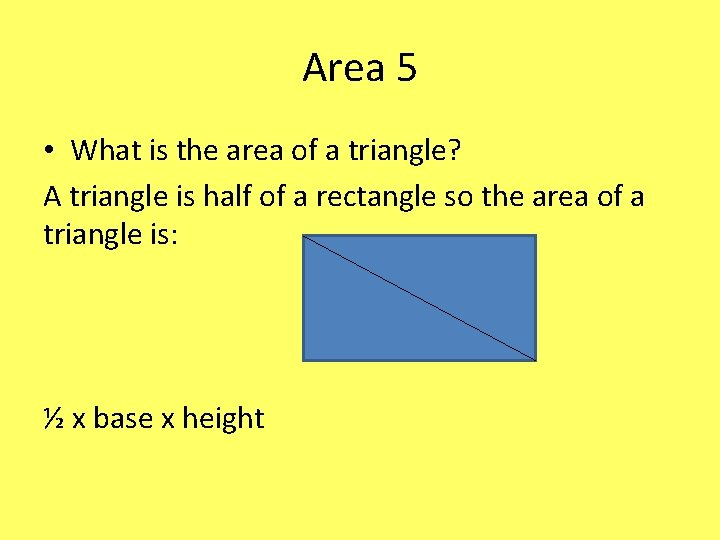Area 5 • What is the area of a triangle? A triangle is half of a rectangle so the area of a triangle is: ½ x base x height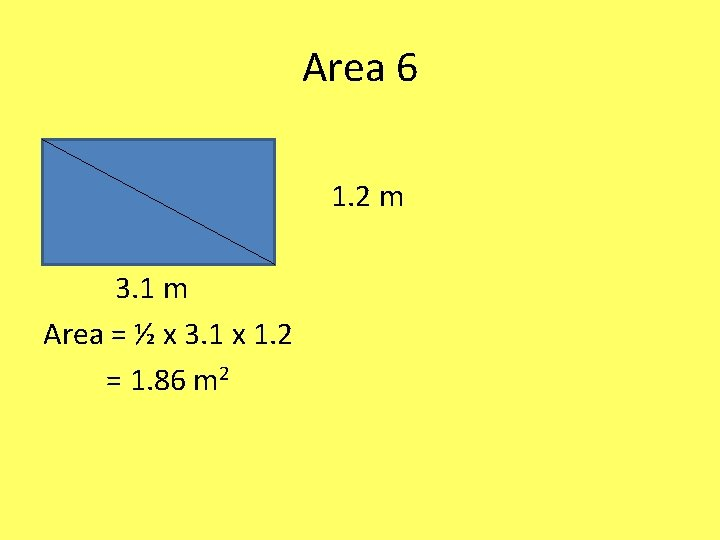Area 6 1. 2 m 3. 1 m Area = ½ x 3. 1 x 1. 2 = 1. 86 m 2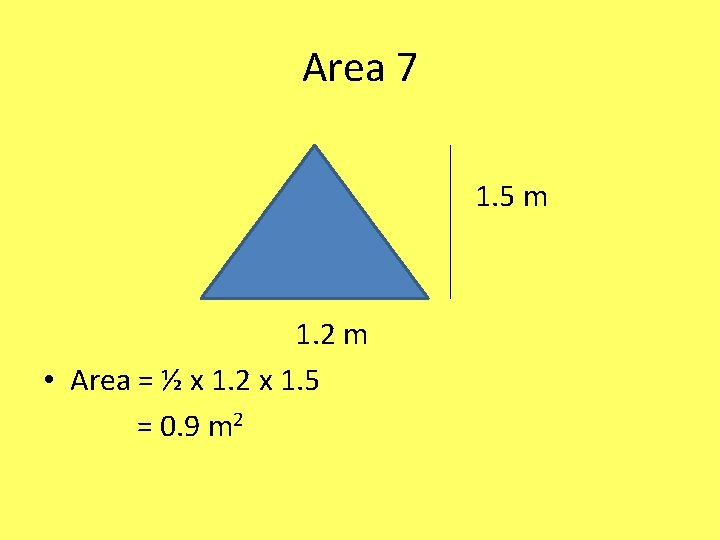Area 7 1. 5 m 1. 2 m • Area = ½ x 1. 2 x 1. 5 = 0. 9 m 2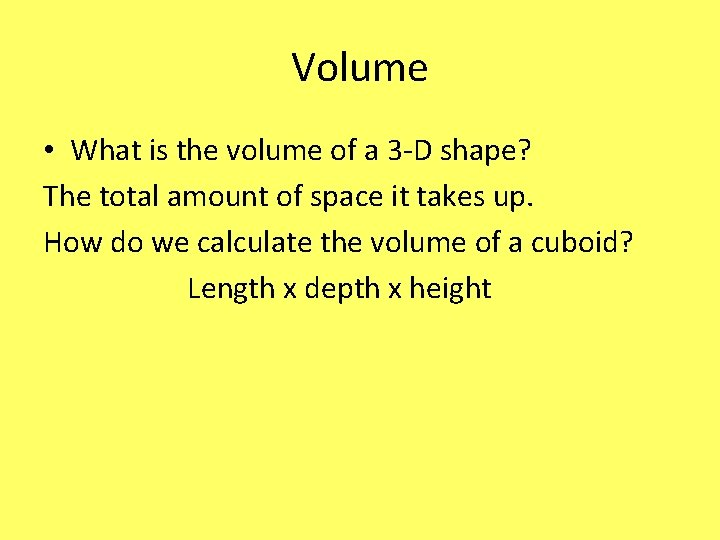Volume • What is the volume of a 3 -D shape? The total amount of space it takes up. How do we calculate the volume of a cuboid? Length x depth x height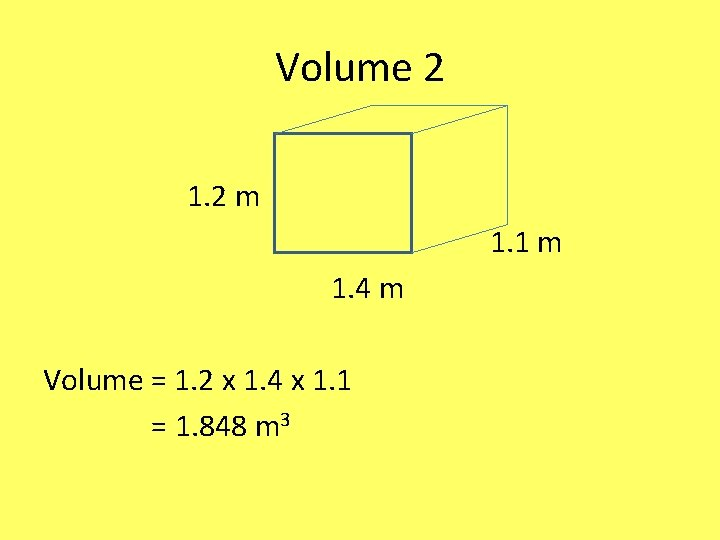Volume 2 1. 2 m 1. 1 m 1. 4 m Volume = 1. 2 x 1. 4 x 1. 1 = 1. 848 m 3